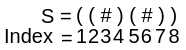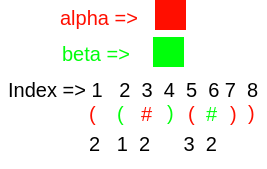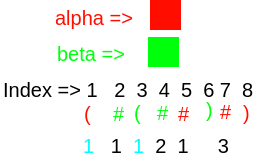Good strings
/

## Algorithms, C++, Depth First Search, Dynamic Programming, Graphs, Implementation

Problem
Editorial
Analytics

There is a string consisting of only three characters (, ) and #.

For Example:-Here, the bracket pairs are 1 -> 8, 2 -> 4, and 5 -> 7 and each bracket pair can have some non - negative value associated with it.

You can categorize the bracket pair and # into two categories alpha and beta. # are also associated with some non-negative values which will be provided in the input.

Now you have to assign the category to each of the characters (i.e. alpha or beta) and non-negative values to the bracket pair such that the given string is the good string.

The good string is the string if the gamma values for each of the bracket pair is equal to Val(Valis provided in the input).

Gamma value is the sum of all the values of the character contained in the bracket pair whose category is the same as the category of the current bracket pair.

For example:-the gamma value for bracket pair 1 -> 8 is  2 (it's own value ) + 2 (value of # at index 3) + 3 (value of bracket pair 5 -> 7) = 6

Now the string to be good the gamma value of each bracket pair should be equal to the values given in the input.

Now you have to assign the categories to the characters(both bracket pair and #) and values to the bracket such that the gamma value of the bracket pair is equal to the Vali (this will be provided in the input).

Now you have to find that, Is it possible to make the string good. If it is possible then print Yes otherwise No.

Input Format:-

The first line of the input contains an integer n(length of the string)

The second line of the input contains the string of length n

Now let the number of # in the string be x, So next x lines will be containing two integers indx and val each, here indx is the index of the # and val is the value corresponding to that #.

Now let the number of bracket pairs in the string be y, So next y lines will be containing 3 non-negative integers start, end, and Val. Here start is the starting index of that bracket pair, the end is the index of the ending of that bracket pair and Val is the expected gamma value of that bracket pair after performing the operations of categorizing the characters and assigning the values to the bracket pairs.

Note:- It is guaranteed that input will be valid.

Output Format:-

Print "Yes" if it is possible to make it the string good otherwise print "No". (without quotes).

Constraints:-

1 <= n <= 2000

1 <= indx <= n

1 <= value of # <= 5000

1 <= starting index of bracket pair < ending index of bracket pair <= n

1 <= Vali <= 50000

SAMPLE INPUT
8
(#(##)#)
2 1
4 2
5 1
7 3
1 8 5
3 6 3


SAMPLE OUTPUT
Yes


ExplanationNote:-

• Black Color numbers are already given in the input
• Blue Color numbers are chosen to make the string good

This is the good string as

the gamma value for bracket pair 1 -> 8 is 1 (It's own) + 1 (# at index 5) + 3 (# at index 7) = 5

the gamma value for bracket pair 3 -> 6 is 1 (It's own) + 2 (# at index 4) = 3

so, both of the bracket pairs have the same value as mentioned in the input. So the string is the good string.

Time Limit: 2.0 sec(s) for each input file.
Memory Limit: 256 MB
Source Limit: 1024 KB

## This Problem was Asked in

Initializing Code Editor...